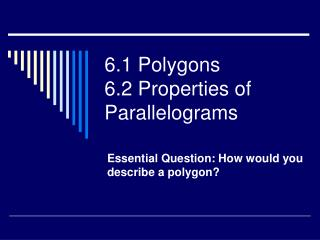DownloadDownload Presentation6.1 Polygons 6.2 Properties of Parallelograms

# 6.1 Polygons 6.2 Properties of Parallelograms

Download Presentation## 6.1 Polygons 6.2 Properties of Parallelograms

- - - - - - - - - - - - - - - - - - - - - - - - - - - E N D - - - - - - - - - - - - - - - - - - - - - - - - - - -
##### Presentation Transcript

1. 6.1 Polygons6.2 Properties of Parallelograms Essential Question: How would you describe a polygon?

2. Polygons • Plane figure formed by three or more sides. • Each endpoint of a side is a vertex. • To name a polygon, list its vertices in order.

3. Polygons are named by the number of sides they have:

4. Describing Polygons • Convex • Concave; (Hint: side is caved in)

5. Equilateral • Equiangular • Regular – all angles and sides are the same. *do #1-13 from overhead

6. Diagonal – segment that joins two vertices.

8. Assignment • P.325 #4-20, 24-26, 37-39, 41-45

9. 6.2 Parallelograms • Parallelogram- quadrilateral with both pairs of opposite sides parallel

10. 4 Properties of Parallelograms • If a quadrilateral is a parallelogram, then its opposite sides are congruent.

11. If a quadrilateral is a parallelogram, then its opposite angles are congruent.

12. If a quadrilateral is a parallelogram, then its consecutive angles are supplementary.

13. If a quadrilateral is a parallelogram, then its diagonals bisect each other.

14. Examples: Using Properties of Parallelograms:

15. Assignment • Complete #1-21 from overhead • P. 333 #2-37 depending on time